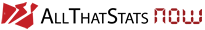# Income Quintile Share Ratio

## Definition

The "income quintile share ratio" (also called the "S80/S20 ratio") is calculated as the ratio of the total income received by the 20% of the population with the highest income (= 1st or top quintile) to that income received by the 20% of the population with the lowest (= 5th or bottom quintile). In other words: The "income quintile share ratio" is nothing else than the annual income of the top 20% of the population expressed in the number of years the lowest 20% of the population have to work in order to archieve the same income result.

## Sources

The "Income Quintile Share Ratio" has been calculated by various source, e.g. by Eurostat. Examples for some relevant databases: Inequality of income distribution S80/S20 income quintile share ratio - EU-SILC survey
S80/S20 income quintile share ratio by sex and selected age group - EU-SILC survey
Inequality of income distribution

# Related ATS topics

Income Distribution: GINI Index

All graphics and tables are created with